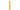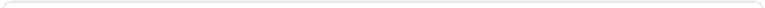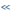Sign InContactsupport: Customer Portal Focused on delivering choice, investment protection and flexibility to organizations with valuable COBOL assets

Veryant Knowledge Base
Home > All Categories > Data Access > COBOL/ESQL and Veryant ESQL Generator > How is READ NEXT implemented in isCOBOL ESQL Generator (EasyDB)?How is READ NEXT implemented in isCOBOL ESQL Generator (EasyDB)?
The START statement creates a cursor, and READ NEXT/PREVIOUS fetches the cursor. Using the isCOBOL debugger, you can step into the READ NEXT and the source code for the bridge programs generated by edbiis.exe to give you a better understanding of how this works.

By default, each elementary item maps to a separate column in the RDBMS. In order to read rows ordered by a composite key, the generated code uses multiple cursors successively with different where clauses.

(Note that you can use the EFD USE-GROUP directive to instruct the isCOBOL ESQL Generator to store a composite key in a single column in the table. See the isCOBOL APS Documentation : isCOBOL ESQL Generator : Using EFD directives : USE GROUP Directive for more information.)

Here is an example:

With the following FD key:

FD ACC.
01 ACC-REC.
05 ACC-KEY.
10 ACC-ID PIC 9(6).
10 ACC-TY PIC 9(3).
10 ACC-CU PIC 9(3).
10 ACC-BR PIC 9(3).
10 ACC-IN-CD PIC 9(2).

If you look at the generated code you will see the following WHERE clauses:

where (ACC_ID = ? and ACC_TY = ? and ACC_CU = ? and ACC_BR = ? and ACC_IN_CD >= ?)

where (ACC_ID = ? and ACC_TY = ? and ACC_CU = ? and ACC_BR > ?)

where (ACC_ID = ? and ACC_TY = ? and ACC_CU > ?)

where (ACC_ID = ? and ACC_TY > ?)

where (ACC_ID > ?)

so that if ACC-KEY is 00000100200300405 then the code for START ACC KEY NOT < ACC-KEY followed by a READ NEXT loop first uses:

where (ACC_ID = 1 and ACC_TY = 2 and ACC_CU = 3 and ACC_BR = 4 and ACC_IN_CD >= 5) order by ACC_ID, ACC_TY, ACC_BR, ACC_IN_CD

and after returning all of the rows in that result set (or if the result set it empty), then the code uses

where (ACC_ID = 1 and ACC_TY = 2 and ACC_CU = 3 and ACC_BR > 4) order by ACC_ID, ACC_TY, ACC_BR, ACC_IN_CD

and after returning all of the rows in that result set (or if the result set it empty), then the code uses

where (ACC_ID = 1 and ACC_TY = 2 and ACC_CU > 3) order by ACC_ID, ACC_TY, ACC_BR, ACC_IN_CD

and after returning all of the rows in that result set (or if the result set it empty), then the code uses

where (ACC_ID = 1 and ACC_TY > 2) order by ACC_ID, ACC_TY, ACC_BR, ACC_IN_CD

and after returning all of the rows in that result set (or if the result set it empty), then the code uses

where (ACC_ID > 1) order by ACC_ID, ACC_TY, ACC_BR, ACC_IN_CD

Authored by: Veryant Support This question has been viewed 4585 times so far.
Click Here to View all the questions in COBOL/ESQL and Veryant ESQL Generator category.File Attachments
There are no attachment file(s) related to this question.User CommentsRelated QuestionsAdditional Information Article Number: 71 Created: 2009-09-02 12:39 PM Rating:Article OptionsPrint this QuestionEmail Question to FriendExport to PDF FileExport to MS WordSubscribe to ArticleSearch Knowledge BaseKnowledge Base Home